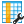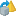You are viewing the RapidMiner Studio documentation for version 9.2 - Check here for latest version

#Principal Component Analysis (Kernel) (RapidMiner Studio Core)

## Synopsis

This operator performs Kernel Principal Component Analysis (PCA) which is a non-linear extension of PCA.

## Description

Kernel principal component analysis (kernel PCA) is an extension of principal component analysis (PCA) using techniques of kernel methods. Using a kernel, the originally linear operations of PCA are done in a reproducing kernel Hilbert space with a non-linear mapping. By the use of integral operator kernel functions, one can efficiently compute principal components in high-dimensional feature spaces, related to input space by some nonlinear map. The result will be the set of data points in a non-linearly transformed space. Please note that in contrast to the usual linear PCA the kernel variant also works for large numbers of attributes but will become slow for large number of examples.

RapidMiner provides the Principal Component Analysis operator for applying linear PCA. Principal Component Analysis is a mathematical procedure that uses an orthogonal transformation to convert a set of observations of possibly correlated attributes into a set of values of uncorrelated attributes called principal components. This transformation is defined in such a way that the first principal component's variance is as high as possible (accounts for as much of the variability in the data as possible), and each succeeding component in turn has the highest variance possible under the constraint that it should be orthogonal to (uncorrelated with) the preceding components.

## Differentiation

###Principal Component Analysis

Kernel principal component analysis (kernel PCA) is an extension of principal component analysis (PCA) using techniques of kernel methods. In contrast to the usual linear PCA the kernel variant also works for large numbers of attributes but will become slow for large number of examples.

## Input

•example set input (IOObject)

This input port expects an ExampleSet. It is output of the Retrieve operator in the attached Example Process. The output of other operators can also be used as input. It is essential that meta data should be attached with the data for the input because attributes are specified in their meta data. The Retrieve operator provides meta data along with the data. Please note that this operator cannot handle nominal attributes; it works on numerical attributes.

## Output

•example set output (IOObject)

The kernel-based Principal Component Analysis is performed on the input ExampleSet and the resultant ExampleSet is delivered through this port.

•original (IOObject)

The ExampleSet that was given as input is passed without changing to the output through this port. This is usually used to reuse the same ExampleSet in further operators or to view the ExampleSet in the Results Workspace.

•preprocessing model (Preprocessing Model)

This port delivers the preprocessing model, which has the information regarding the parameters of this operator in the current process.

## Parameters

• kernel_typeThe type of the kernel function is selected through this parameter. Following kernel types are supported: dot, radial, polynomial, neural, anova, epachnenikov, gaussian combination, multiquadric
• dot: The dot kernel is defined by k(x,y)=x*y i.e. it is inner product of x and y.
• radial: The radial kernel is defined by exp(-g ||x-y||^2) where g is the gamma, it is specified by the kernel gamma parameter. The adjustable parameter gamma plays a major role in the performance of the kernel, and should be carefully tuned to the problem at hand.
• polynomial: The polynomial kernel is defined by k(x,y)=(x*y+1)^d where d is the degree of polynomial and it is specified by the kernel degree parameter. The polynomial kernels are well suited for problems where all the training data is normalized.
• neural: The neural kernel is defined by a two layered neural net tanh(a x*y+b) where a is alpha and b is the intercept constant. These parameters can be adjusted using the kernel a and kernel b parameters. A common value for alpha is 1/N, where N is the data dimension. Note that not all choices of a and b lead to a valid kernel function.
• anova: The anova kernel is defined by raised to power d of summation of exp(-g (x-y)) where g is gamma and d is degree. gamma and degree are adjusted by the kernel gamma and kernel degree parameters respectively.
• epachnenikov: The epachnenikov kernel is this function (3/4)(1-u2) for u between -1 and 1 and zero for u outside that range. It has two adjustable parameters kernel sigma1 and kernel degree.
• gaussian_combination: This is the gaussian combination kernel. It has adjustable parameters kernel sigma1, kernel sigma2 and kernel sigma3.
• multiquadric: The multiquadric kernel is defined by the square root of ||x-y||^2 + c^2. It has adjustable parameters kernel sigma1 and kernel sigma shift.
Range: selection
• kernel_gammaThis is the kernel parameter gamma. This is only available when the kernel type parameter is set to radial or anova. Range: real
• kernel_sigma1This is the kernel parameter sigma1. This is only available when the kernel type parameter is set to epachnenikov, gaussian combination or multiquadric. Range: real
• kernel_sigma2This is the kernel parameter sigma2. This is only available when the kernel type parameter is set to gaussian combination. Range: real
• kernel_sigma3This is the kernel parameter sigma3. This is only available when the kernel type parameter is set to gaussian combination. Range: real
• kernel_shiftThis is the kernel parameter shift. This is only available when the kernel type parameter is set to multiquadric. Range: real
• kernel_degreeThis is the kernel parameter degree. This is only available when the kernel type parameter is set to polynomial, anova or epachnenikov. Range: real
• kernel_aThis is the kernel parameter a. This is only available when the kernel type parameter is set to neural. Range: real
• kernel_bThis is the kernel parameter b. This is only available when the kernel type parameter is set to neural. Range: real

## Tutorial Processes

### Introduction to the Principal Component Analysis (Kernel) operator

The 'Polynomial' data set is loaded using the Retrieve operator. A breakpoint is inserted here so that you can have a look at the ExampleSet. You can see that the ExampleSet has 5 regular attributes. The Principal Component Analysis (Kernel) operator is applied on this ExampleSet with default values of all parameters. The kernel type parameter is set to 'radial' and the kernel gamma parameter is set to 1.0. The resultant ExampleSet can be seen in the Results Workspace. You can see that this ExampleSet has a different set of attributes.